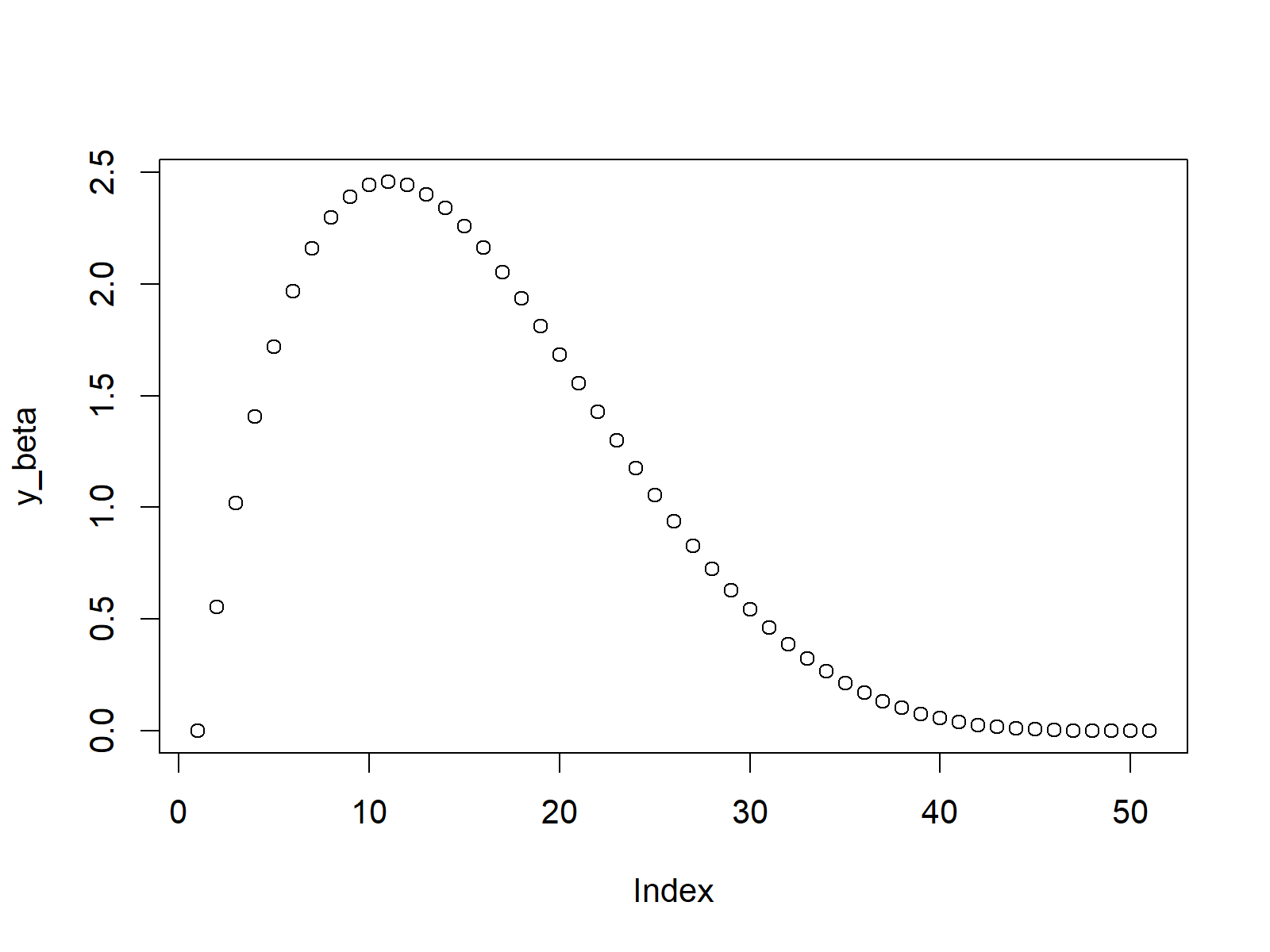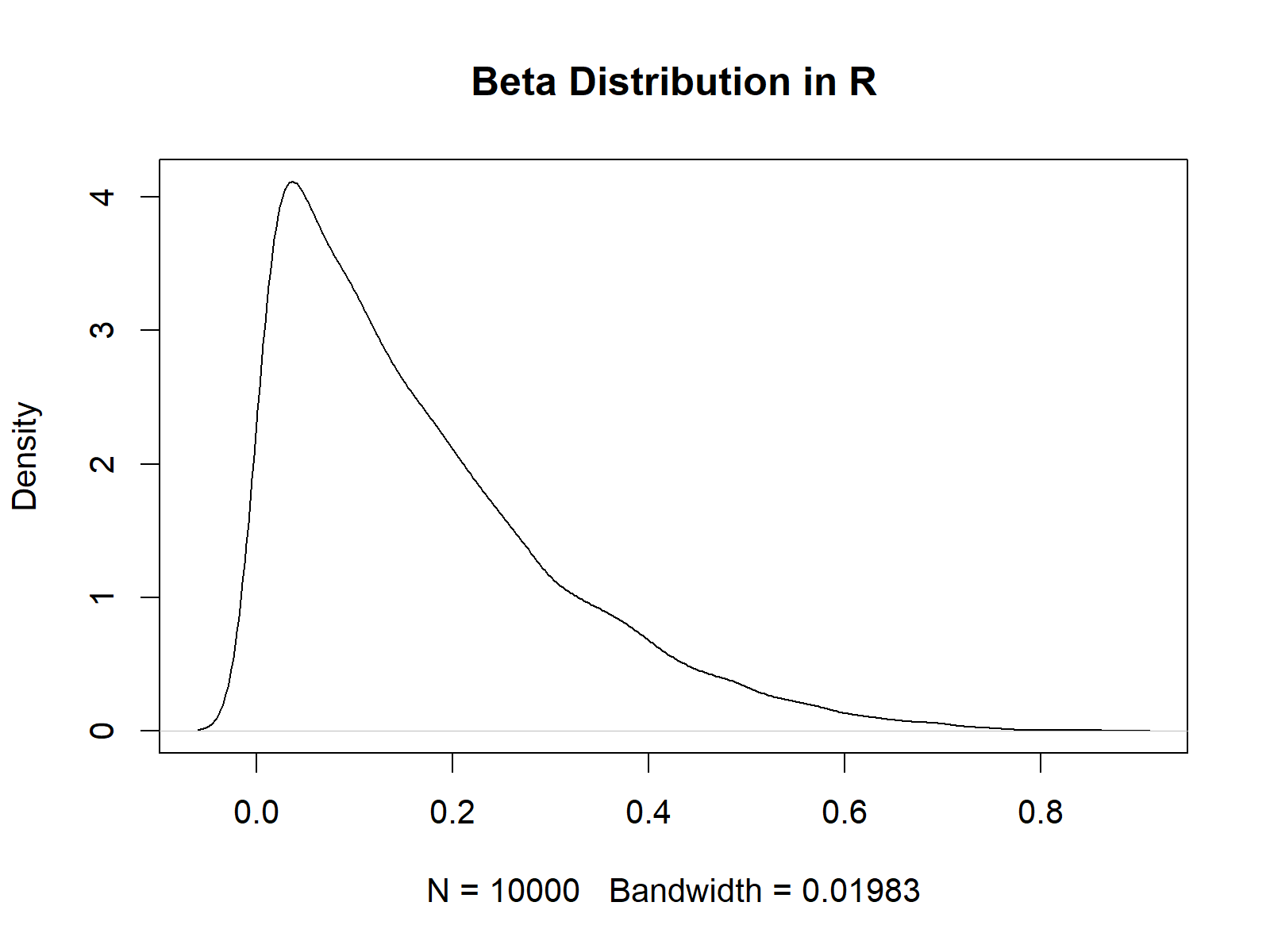# Beta Distribution in R (4 Examples) | dbeta, pbeta, qbeta & rbeta Functions

This article shows how to use the beta functions in R programming.

The content of the page looks as follows:

## Example 1: Beta Density in R (dbeta Function)

The dbeta R command can be used to return the corresponding beta density values for a vector of quantiles.

Let’s create such a vector of quantiles in R:

`x_beta <- seq(0, 1, by = 0.02)                     # Specify x-values for beta function`

Now, we can apply the dbeta function to return the values of the beta density that correspond to our input vector and the two shape parameters shape1 and shape2 (i.e. 2 and 5):

`y_beta <- dbeta(x_beta, shape1 = 2, shape2 = 5)    # Apply beta function`

We can also create a graphic in R, which shows our previously created values:

`plot(y_beta)                                       # Plot beta values`Figure 1: Beta Density in R.

## Example 2: Beta Distribution Function (pbeta Function)

In the second example, we will draw a cumulative distribution function of the beta distribution. For this task, we also need to create a vector of quantiles (as in Example 1):

`x_pbeta <- seq(0, 1, by = 0.02)                    # Specify x-values for pbeta function`

This vector of quantiles can now be inserted into the pbeta function:

`y_pbeta <- pbeta(x_pbeta, shape1 = 1, shape2 = 5)  # Apply pbeta function`

The output is shown in the following graph:

`plot(y_pbeta)                                      # Plot pbeta values`Figure 2: Cumulative Distribution Function of Beta Distribution.

## Example 3: Beta Quantile Function (qbeta Function)

The R programming language also provides the possibility to return the values of the beta quantile function. This time we need to create sequence of probabilities as input:

`x_qbeta <- seq(0, 1, by = 0.02)                    # Specify x-values for qbeta function`

These probabilities can now be inserted into the qbeta function:

`y_qbeta <- qbeta(x_qbeta, shape1 = 1, shape2 = 5)  # Apply qbeta function`

The following R code produces the corresponding R plot:

`plot(y_qbeta)                                      # Plot qbeta values`Figure 3: Beta Quantile Function.

## Example 4: Random Number Generation (rbeta Function)

In case we want to generate random numbers from the beta density, we need to set a seed and specify our desired sample size first:

```set.seed(13579)                                    # Set seed for reproducibility
N <- 10000                                         # Specify sample size```

Now, we can use the rbeta function to simulate a set of random numbers drawn from the beta distribution:

```y_rbeta <- rbeta(N, shape1 = 1, shape2 = 5)        # Draw N beta distributed values
y_rbeta                                            # Print values to RStudio console
# 0.2311784008 0.0305951096 0.0527656109 0.1289216944 0.2317310221 0.0557187181 0.0855674210 0.4125831741 0.2879114830...```

The RStudio console is showing the output of the rbeta function. Let’s plot these values in a density plot:

```plot(density(y_rbeta),                             # Plot of randomly drawn beta density
main = "beta Distribution in R")```Figure 4: Random Numbers Drawn from Beta Density.

## Video & Further Resources

In case you need more information on the R programming codes of this article, I can recommend to have a look at the following video of my YouTube channel. I show the R syntax of this post in the video:

You could also read the other articles on probability distributions and the simulation of random numbers in R:

Also, you could have a look at the related tutorials on this website.

Summary: In this tutorial, I illustrated how to calculate and simulate a beta distribution in R programming. Tell me about it in the comments below, in case you have any further comments or questions.

Subscribe to the Statistics Globe Newsletter

•Huy Truong
June 25, 2022 6:56 pm

Hello, could you please explain what “a vector of quantiles” mean? Thanks!

•June 27, 2022 6:46 am

Hey Huy,

A quantile cuts the values in a data object into a certain number of equal groups. Have a look at this Wikipedia article for more details.

In the present tutorial, I’m defining 50 cut-points using this code:

`x_beta <- seq(0, 1, by = 0.02)`

x_beta is our vector of quantiles.

I’m using this vector to calculate the corresponding distribution values for these cut-points.

I hope that helps!

Joachim

•Sam
August 5, 2022 5:41 pm

Great work. I need help, I am trying to do meta analysis of proportion but finding it difficult to get the right functions.

•August 5, 2022 6:43 pm